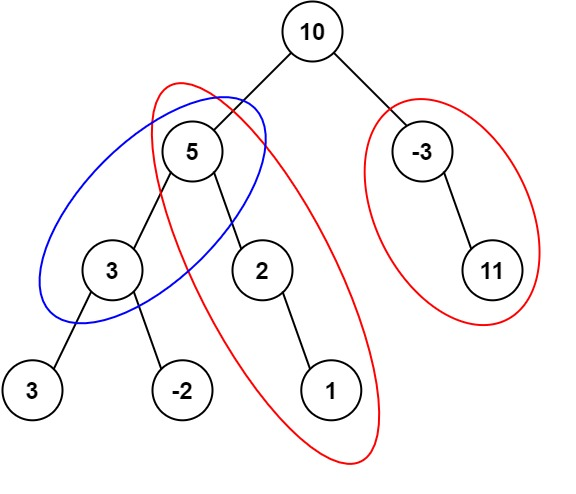## C# || How To Get The Number Of Binary Tree Paths Equal To Path Sum Using C#The following is a module with functions which demonstrates how to get the number of binary tree paths equal to path sum using C#.

1. Number Of Path Sum Paths – Problem Statement

Given the root of a binary tree and an integer targetSum, return the number of paths where the sum of the values along the path equals targetSum.

The path does not need to start or end at the root or a leaf, but it must go downwards (i.e., traveling only from parent nodes to child nodes).

Example 1:``` Input: root = [10,5,-3,3,2,null,11,3,-2,null,1], targetSum = 8 Output: 3 Explanation: The paths that sum to 8 are shown. ```

Example 2:

``` Input: root = [5,4,8,11,null,13,4,7,2,null,null,5,1], targetSum = 22 Output: 3 ```

2. Number Of Path Sum Paths – Solution

The following is a solution which demonstrates how to get the number of binary tree paths equal to path sum.

The main idea here is that the sum at each level for each path is calculated. When the next level is explored, the value at the previous level is summed together with the node value at the current level.

A map dictionary is used to keep track of the sum at each level. If the prefix sum at the previous level is enough to equal the target path sum at the current level, the result count is incremented.

``` 2. Number Of Path Sum Paths - Solution C# // ============================================================================ // Author: Kenneth Perkins // Date: Oct 18, 2021 // Taken From: http://programmingnotes.org/ // File: Solution.cs // Description: Determines how to get the number of tree paths equal to path sum // ============================================================================ /** * Definition for a binary tree node. * public class TreeNode { * public int val; * public TreeNode left; * public TreeNode right; * public TreeNode(int val=0, TreeNode left=null, TreeNode right=null) { * this.val = val; * this.left = left; * this.right = right; * } * } */ public class Solution { private int result = 0; private Dictionary<int, int> sumFrequency = new Dictionary<int, int>(); public int PathSum(TreeNode root, int targetSum) { sumFrequency = 1; Search(root, targetSum, 0); return result; } public void Search(TreeNode node, int targetSum, int currentSum) { if (node == null) { return; } // Determine the current sum currentSum = currentSum + node.val; // Get the path prefix sum var prefixSum = currentSum - targetSum; if (sumFrequency.ContainsKey(prefixSum)) { result += sumFrequency[prefixSum]; } // Increment the number of times this prefix has been seen sumFrequency[currentSum] = (sumFrequency.ContainsKey(currentSum) ? sumFrequency[currentSum] : 0) + 1; // Keep exploring along branches finding the target sum Search(node.left, targetSum, currentSum); Search(node.right, targetSum, currentSum); // Remove value of this prefixSum (path's been explored) --sumFrequency[currentSum]; } }// http://programmingnotes.org/ 1234567891011121314151617181920212223242526272829303132333435363738394041424344454647484950515253545556 // ============================================================================//    Author: Kenneth Perkins//    Date:   Oct 18, 2021//    Taken From: http://programmingnotes.org///    File:  Solution.cs//    Description: Determines how to get the number of tree paths equal to path sum// ============================================================================/** * Definition for a binary tree node. * public class TreeNode { *     public int val; *     public TreeNode left; *     public TreeNode right; *     public TreeNode(int val=0, TreeNode left=null, TreeNode right=null) { *         this.val = val; *         this.left = left; *         this.right = right; *     } * } */public class Solution {    private int result = 0;    private Dictionary<int, int> sumFrequency = new Dictionary<int, int>();     public int PathSum(TreeNode root, int targetSum) {        sumFrequency = 1;        Search(root, targetSum, 0);        return result;    }     public void Search(TreeNode node, int targetSum, int currentSum) {        if (node == null) {            return;        }         // Determine the current sum        currentSum = currentSum + node.val;         // Get the path prefix sum        var prefixSum = currentSum - targetSum;         if (sumFrequency.ContainsKey(prefixSum)) {            result += sumFrequency[prefixSum];        }         // Increment the number of times this prefix has been seen        sumFrequency[currentSum] = (sumFrequency.ContainsKey(currentSum) ? sumFrequency[currentSum] : 0) + 1;         // Keep exploring along branches finding the target sum        Search(node.left, targetSum, currentSum);        Search(node.right, targetSum, currentSum);         // Remove value of this prefixSum (path's been explored)        --sumFrequency[currentSum];    }}// http://programmingnotes.org/ ```

QUICK NOTES:
The highlighted lines are sections of interest to look out for.

The code is heavily commented, so no further insight is necessary. If you have any questions, feel free to leave a comment below.

Once compiled, you should get this as your output for the example cases:

``` 3 3 ```